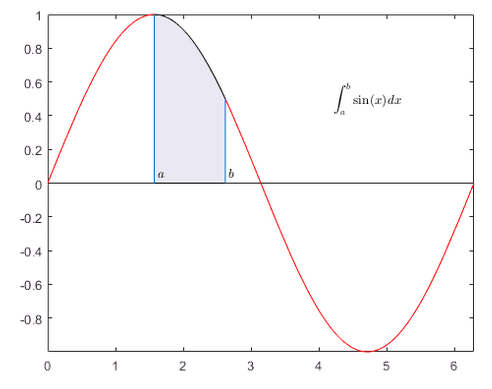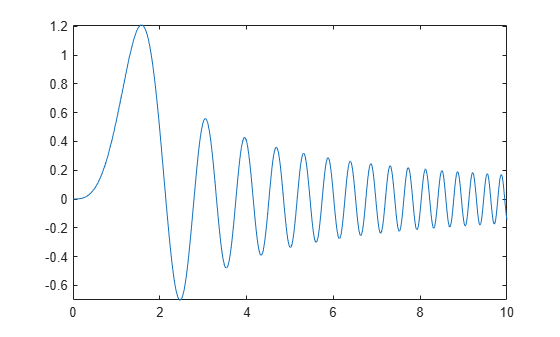Documentation

### This is machine translation

Mouseover text to see original. Click the button below to return to the English version of the page.

Note: This page has been translated by MathWorks. Click here to see
To view all translated materials including this page, select Country from the country navigator on the bottom of this page.

## Integration

This example shows how to compute definite integrals using Symbolic Math Toolbox™.### Definite Integral

Show that the definite integral $\underset{a}{\overset{b}{\int }}f\left(x\right)dx$ for $f\left(x\right)=sin\left(x\right)$ on $\left[\frac{\pi }{2},\frac{3\pi }{2}\right]$ is 0.

```syms x int(sin(x),pi/2,3*pi/2)```
`ans = $0$`

### Definite Integrals in Maxima and Minima

To maximize $F\left(a\right)={\int }_{-a}^{a}\mathrm{sin}\left(ax\right)\mathrm{sin}\left(x/a\right)\phantom{\rule{0.16666666666666666em}{0ex}}dx$ for $a\ge 0$, first, define the symbolic variables and assume that $a\ge 0$:

```syms a x assume(a >= 0);```

Then, define the function to maximize:

`F = int(sin(a*x)*sin(x/a),x,-a,a)`
```F =  ```

Note the special case here for $a=1$. To make computations easier, use `assumeAlso` to ignore this possibility (and later check that $a=1$ is not the maximum):

```assumeAlso(a ~= 1); F = int(sin(a*x)*sin(x/a),x,-a,a)```
```F =  $\frac{2 a \left(\mathrm{sin}\left({a}^{2}\right) \mathrm{cos}\left(1\right)-{a}^{2} \mathrm{cos}\left({a}^{2}\right) \mathrm{sin}\left(1\right)\right)}{{a}^{4}-1}$```

Create a plot of $F$ to check its shape:

`fplot(F,[0 10])`Use `diff` to find the derivative of $F$ with respect to $a$:

`Fa = diff(F,a)`
```Fa =  ```

The zeros of $Fa$ are the local extrema of $F$:

```hold on fplot(Fa,[0 10]) grid on```The maximum is between 1 and 2. Use `vpasolve` to find an approximation of the zero of $Fa$ in this interval:

`a_max = vpasolve(Fa,a,[1,2])`
`a_max = $1.5782881585233198075558845180583$`

Use `subs` to get the maximal value of the integral:

`F_max = subs(F,a,a_max)`
`F_max = $0.36730152527504169588661811770092 \mathrm{cos}\left(1\right)+1.2020566879911789986062956284113 \mathrm{sin}\left(1\right)$`

The result still contains exact numbers $\mathrm{sin}\left(1\right)$ and $\mathrm{cos}\left(1\right)$. Use `vpa` to replace these by numerical approximations:

`vpa(F_max)`
`ans = $1.2099496860938456039155811226054$`

Check that the excluded case $a=1$ does not result in a larger value:

`vpa(int(sin(x)*sin(x),x,-1,1))`
`ans = $0.54535128658715915230199006704413$`

### Multiple Integration

Numerical integration over higher dimensional areas has special functions:

`integral2(@(x,y) x.^2-y.^2,0,1,0,1)`
```ans = 0 ```

There are no such special functions for higher-dimensional symbolic integration. Use nested one-dimensional integrals instead:

```syms x y int(int(x^2-y^2,y,0,1),x,0,1)```
`ans = $0$`

### Line Integrals

Define a vector field `F` in 3D space:

```syms x y z F(x,y,z) = [x^2*y*z, x*y, 2*y*z];```

Next, define a curve:

```syms t ux(t) = sin(t); uy(t) = t^2-t; uz(t) = t;```

The line integral of `F` along the curve `u` is defined as $\int f\cdot du=\int f\left(ux\left(t\right),uy\left(t\right),uz\left(t\right)\right)\cdot \frac{du}{dt}\phantom{\rule{0.16666666666666666em}{0ex}}dt$, where the $\cdot$ on the right-hand-side denotes a scalar product.

Use this definition to compute the line integral for $t$ from $\left[0,1\right]$

`F_int = int(F(ux,uy,uz)*diff([ux;uy;uz],t),t,0,1)`
```F_int =  $\frac{19 \mathrm{cos}\left(1\right)}{4}-\frac{\mathrm{cos}\left(3\right)}{108}-12 \mathrm{sin}\left(1\right)+\frac{\mathrm{sin}\left(3\right)}{27}+\frac{395}{54}$```

Get a numerical approximation of this exact result:

`vpa(F_int)`
`ans = $-0.20200778585035447453044423341349$`

#### Mathematical Modeling with Symbolic Math Toolbox

Get examples and videos